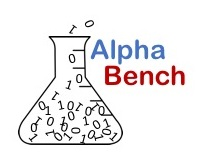## Numpy for Beginners

Numpy is the fundamental scientific platform library for Python. In sone cases you will use its powerful array data structure directly, and in other case you won't even know numpy is on the job. This is because the numpy array underpins many common libraries used when working with data including Matplotlib and Pandas.

## Numpy Tutorial

The two videos below walk through numpy basics including, creating numpy arrays, numpy special placeholder arrays, indexing elements, "columns" and "rows" in multidimensional arrays. You will also see how boradcast, or vectorized, mathematical operations work in numpy as well as common statistical functions and data summary techniques.